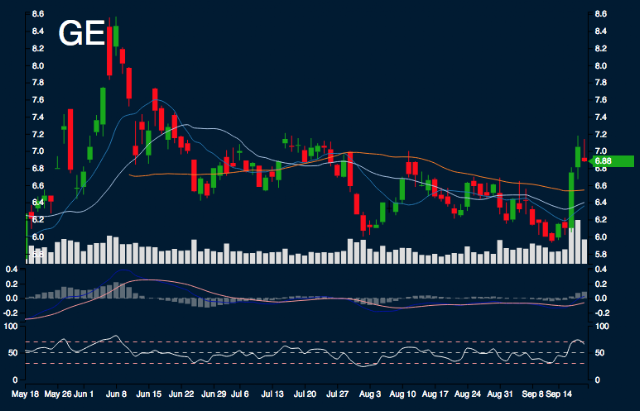#GE Report

Sample Size: 7; Percent Error: 5%

## Current Trend Performance

GE is -3.78% from monthly high, 13.52% from monthly low, 8.18% from 10 day simple moving average, 7.51% from 20 day simple moving average, and 5.12% from 50 day simple moving average.

## Forecast Ranges

Day 1:
The highs are 6.97, the lows are 6.79, and the median is 6.88. 33.33% to decrease between -2.64% - 0%. 66.67% to increase between 0% - 2.64%.
Day 2:
The highs are 6.98, the lows are 6.83, and the median is 6.91. 42.86% to decrease between -2.64% - 0%. 57.14% to increase between 0% - 2.64%.
Day 3:
The highs are 7.03, the lows are 6.81, and the median is 6.92. 28.57% to decrease between -2.64% - 0%. 71.43% to increase between 0% - 2.64%.
Day 4:
The highs are 7.1, the lows are 6.68, and the median is 6.89. 14.29% to decrease between -5.28% - -2.64%. 28.57% to decrease between -2.64% - 0%. 42.86% to increase between 0% - 2.64%. 14.29% to increase between 2.64% - 5.28%.
Day 5:
The highs are 7.11, the lows are 6.69, and the median is 6.9. 14.29% to decrease between -5.28% - -2.64%. 28.57% to decrease between -2.64% - 0%. 42.86% to increase between 0% - 2.64%. 14.29% to increase between 2.64% - 5.28%.
Day 6:
The highs are 7.16, the lows are 6.64, and the median is 6.9. 14.29% to decrease between -5.28% - -2.64%. 57.14% to decrease between -2.64% - 0%. 14.29% to increase between 0% - 2.64%. 14.29% to increase between 2.64% - 5.28%.
Day 7:
The highs are 7.12, the lows are 6.62, and the median is 6.87. 28.57% to decrease between -5.28% - -2.64%. 28.57% to decrease between -2.64% - 0%. 14.29% to increase between 0% - 2.64%. 28.57% to increase between 2.64% - 5.28%.

## Summary

SMA: Simple Moving Average

In next 5 days, there is possible support range between 6.7 and 6.8. There is possible resistance range between 6.98 and 7.06. Areas of uncertainty are beyond 6.6 and beyond 7.14. Minimum movement range is 2.64%.

Research conducted with Nforecast.

This is not a recommendation to buy, sell, or hold. This research is for educational, informational, and entertainment purposes. You should not construe any data or information presented by members on this blog or Nforecast LLC as investment, tax, legal, financial or other advice.